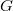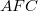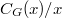﻿

### A note on groups with restrictions on centralizers of in nite index

#### Abstract

A group$G$ is said to be an {\it$AFC$-group} if for each element$x$ of$G$, either$x$ has finitely many conjugates or the factor group$C_G(x)/\cyc x$ is finite. In this survey article some results concerning$AFC$-groups and minimal-non-$AFC$ groups are collected

DOI Code: 10.1285/i15900932v36suppl1p1

Keywords: $AFC$-group; minimal-non-$AFC$ group; $FC$-centre

Full Text: PDF